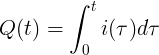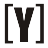# Electric Charge (Q)

Definition of Electric charge is a basic charge carried by basic particles, causing the elementary particles to experience magnetic force.

The electrical charge of a basic particle can be positive and negative. If two objects have the same charge it will reject and the two objects will attract if they have different types of charge.

Basic and subatomic particles such as electrons and protons have an electric charge. Negatively charged electrons and positively charged protons.

## What is Electric Charge?

Electric charge is the basic charge possessed by an object, which makes it experience force on other objects that are nearby and also have an electric charge.

The Q symbol is often used to describe electric charges. The International Unit System (SI) of unit Q is coulomb, which is 6.24 x 1018 basic charge.

### Electric Charge Type

Positive Electric Charge (Proton)
According to Benjamin Franklin, Positive Electric Charge is generally mutually repelling with an object that is charged, and in this case it occurs because the positive charge is similar so that it will act against each other rejecting it.

Negative Electric Charge (Electrons)
According to Benjamin Franklin, Negative Electric Charge on an object can be ascertained if there are objects that have a negative charge and repel each other with plastic that has a charge, it can be ascertained that the charge of the object is negative.

`1 electron charge = -1,6.10-19 coulomb`
`1 proton charge = + 1.6.10-19 coulomb`

The electrical charge of an object is determined by the number of protons and electrons contained in the object.

If an object is excess electron = lack of proton (Σ electron> Σ Proton), then the object is negatively charged
If an object lacks electrons = excess protons (Σ electrons <Σ Proton), then the object is positively charged
If the number of electrons = number of protons (Σ protons = Σ electrons) then the object is not charged (neutral charge)

Properties of Electric Charge

• Similar electric charges will repel each other and non-similar loads will attract each other.
• Electric charge is the principal physical quantity measured in coulomb units symbolized by (C). One coulomb is 6.24 x 1018 e (e = proton charge). So that the sea contained by the proton is 1.602 x 10-19 coulombs. Electrons have the same charge as protons but different types (-) 1,602 x 10-19 coulombs.
• Electric charge has a law of conservation of charge. The force generated by two charges has the same character as the gravitational force which has two objects with a certain mass. The force between loads is also conservative and centralized.

### Electric Charge Formula

The formula that applies in electric charge is a formula that is mathematicized from the coulomb law. Coulomb’s law was discovered by Charles Augustin de Coulomb at the end of the 18th century. The sound of Coulomb’s Law is:

“The attractive force or resistive repulsion between two electric charges is proportional to the charges and inversely proportional to the square of distance separating the two charges.”

Then systematically formulated with:
constant current

`Q = I * t`
```Q = electric charge in coulombs [C]
I = current in amperes [A]
r = distance between the two loads
t = time period in seconds [s]```

momentary current```Q = electric charge in coulombs [C]

i(t) = momentary current in amperes [A]

t = time period in seconds [s]```YoosFuhl.com Would you like to receive notifications on latest updates? No Yes